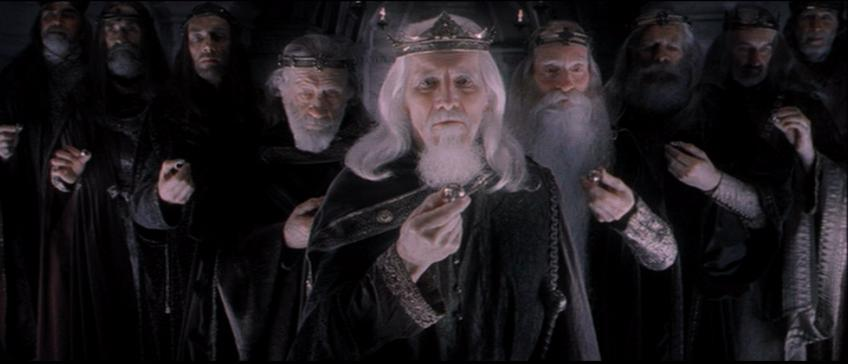# User blogs

Tag search results for: "anton lavey"Sorry for the delay in getting this group going. I recently gave my organization a needed reboot to ensure it went on the path I originally intended.

If anyone is interested, here is the link for my public profile on Facebook since I am on there often.

Throughout the week I will be adding topics and information in here.

Ave Satanas brothers and sisters \m/

Feb 28 '17 ·Chapter HeadIn the Satanic Rituals (THE UNKNOWN KNOWN) Anton LaVey speaks ambiguously and enigmatically about the number 9 being the main number of which to identify with Satan and the ego.

"Despite other's attempts to identify a certain number with Satan (meaning 666), It will be known that Nine is his number. Nine is the number of the Ego, for it always returns to itself. No matter what is done through the most complex multiplication of Nine by any other number, in the final equation nine alone will stand forth." - Anton LaVey, The Satanic Rituals

If you break down the dealings of 9 by employing the use of gematria reduction and Occult numerology, you will see exactly what LaVey was talking about.

Gematria reduction in Occult numerology
Adding any number to 9 reverts back to the original number it was added to, just as adding ego to anything; changes nothing - the status quo endures.

9+1 = 10 - 1+0 = 1

9+2 = 11 - 1+1 = 2

9+3 = 12 - 1+2 = 3

9+4 = 13 - 1+3 = 4

9+5 = 14 - 1+4 = 5

9+6 = 15 - 1+5 = 6

Nine is the number of the Ego, reflecting consciousness in the veil of Paroketh because if you times 9 by any number and it will always persevere as 9.

9x1=9

9x2=18 -1+8=9

9x3=27 -2+7=9

9x4=36 -3+6=9

9x5=45 -4+5=9

9x6=54 -5+4=9

666 - The number of the Beast, the number of Beast consciousness, the veil of the profane. This number always goes back to 9. 9 and 666 are interchangeable and mean the same thing.

666x1= 666 - 6+6+6=18 - 1+8=9

666x2=1332 - 1+3+3+2 =9

666x3=1998 - 1+9+9+8=27 - 2+7 = 9

666x4=2664 - 2+6+6+4=18 1+8 = 9

666x5=3330 - 3+3+3+0=9

666x6=3996 - 3+9+9+6=27 - 2+7 = 9

Jun 9 '16 · Rate: 4.50 · Comments: 8 ·It was said that Anton Lavey was trying to repent to God on his death bed. I think that it is hogwash. He was too passionate about satanism to repent. Maybe he did though...  do any of you know the truth of what happened?
Jun 2 '16 · Comments: 4 · Tags: anton lavey

### Tag Search

Certain features and pages can only be viewed by registered users.